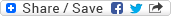## On Chow Rings of Fine Quiver Moduli and Modules over the Cohomological Hall Algebra

### Dateibereich 4113

769,7 KB in einer Datei, zuletzt geändert am 22.01.2018

DateiDateien geändert amGröße
dc1412.pdf22.01.2018 13:01:40769,7 KB

Several classical problems can be reformulated in the language of representations of quivers, for example the classifcation of (an m-tuple of) (d × d)-matrices up to (simultaneous) conjugation. It is possible to give a canonical form for a conjugacy class of a single matrix, for example the Jordan canonical form. However, for a tuple of two or more matrices, such a canonical form cannot be achieved. Thus, instead of trying to fnd a distinct representative of each isomorphism class, we look for a geometric object whose points are in bijection to isomorphism classes of representations of a given quiver. We call such a space a quiver moduli space (or quiver moduli for short). In the author's thesis, we are considering moduli spaces which are varieties. They are constructed using King's application of Mumford's geometric invariant theory to representations of quivers. This theory assures that, after fxing a dimension vector (a numerical invariant) and a stability condition, there exists a variety parametrizing stable representations of the quiver (of the given dimension vector). Our goal is to analyze the cohomology or intersection theory of these moduli spaces.
The frst major result of the thesis (Theorem 3.2.1) answers a question which arises from a theorem of King and Walter. They proved that the Chow ring of a smooth, projective fne quiver moduli space is generated by the Chern classes of its universal family (whose existence is precisely the defnition of a fne moduli space). A natural question to ask is whether there exists a complete set of relations between these generators arising from the given combinatorial data (i.e. the quiver, the dimension vector and the stability condition). The answer is almost positive: We are able to construct a single relation in Chern roots of the universal family associated to every "forbidden" sub-dimension vector. They yield multiple relations in Chern classes called the tautological relations. Together with a "linear" relation, arising from the construction of the universal family, the tautological relations, indeed, give a presentation of the Chow ring of the moduli space.
Both the second and the third main theorem deal with a class of non-projective, smooth fine quiver moduli, namely the non-commutative Hilbert schemes. They parametrize framed stable representations of a quiver with the "unframed" stability condition being trivial. Apart from lacking projectivity, these varieties are particularly well behaved, thus letting us hope to being able to determine its cohomology explicitly.
Based on a cell decomposition of Reineke, we find a basis of the Chow group (with integral coefficients) of the non-commutative Hilbert scheme consisting of monomials in Chern classes of the universal bundle (cf. Corollary 4.4.5). This result is a consequence of Theorem 4.4.1 which proves the transformation matrix, which displays these monomials as a linear combination in the cycles associated to the cell closures, to be upper unitriangular.
Finally, Theorem 5.2.1 relates the cohomology groups (or equally well Chow groups) to Kontsevich's and Soibelman's Cohomological Hall algebra (CoHa). We give the cohomology of noncommutative Hilbert schemes of a quiver the structure of a module over the CoHa of this very quiver (with trivial potential). There is a natural map from the Coha to this module which can easily be seen to be CoHa-linear and surjective. Using Harder-Narasimhan techniques and computations involving fiber bundles, we are able to describe the kernel of this surjection explicitly.

Lesezeichen:
Permalink |Dokumententyp:
Wissenschaftliche Abschlussarbeiten » Dissertation
Fakultäten und Einrichtungen:
Fakultät für Mathematik und Naturwissenschaften » Mathematik und Informatik » Dissertationen
Dewey Dezimal-Klassifikation:
500 Naturwissenschaften und Mathematik » 510 Mathematik » 510 Mathematik
Sprache:
Englisch
Kollektion / Status:
Dissertationen / Dokument veröffentlicht
Dateien geändert am:
22.01.2018
Datum der Promotion:
10.07.2014
Medientyp:
Text